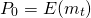# Intertemporal Rate of Substitution

The intertemporal rate of substitution is a concept in finance that helps us to link the long-term growth rate of the economy, investors’ expectations of future consumption, and interest rate to each other. the reason these are interlinked is because investors trade-off between real consumption today and real consumption in the future. To forgo current consumption, investors have to be compensated. That compensation is the interest rate. Their expectations of future consumption depends on what they think their income will be in the future.

On this page, we discuss the intertemporal rate of substitution formula, discuss some key points to keep in mind. It’s very easy to calculate the intertemporal rate of substitution using a spreadsheet.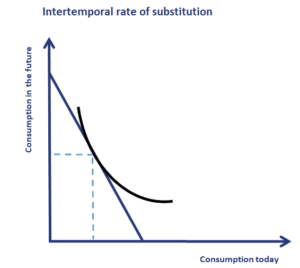## Intertemporal rate of substitution formula

The real risk-free rate of interest derives from the inter-temporal rate of substitution. As we mentioned before, the latter measures the willingness of investors to delay consumption. The formula looks as follows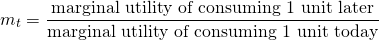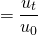An important point to keep in mind is that for a given quantity of consumption, investors always prefer current consumption over future consumption. Thus, mt is generally lower than 1.

The real risk-free rate can then be derived as follows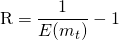This is the risk-free rate of a zero-coupon, inflation-indexed, risk-free bond that will pay 1 USD at time t.

## Important considerations

There are at least three points to keep in mind. First, the higher the utility of current consumption, the higher the real rate. Second, as people become wealthier, the marginal utility of consumption declines. This implies that marginal utility is higher in periods of scarcity. Finally, if investors expect to earn more in the future, their expected marginal utility of future consumption is decreased relative to current consumption.

## Summary

The rate of substitution measures investors’ willingness to delay consumption. Since investors want to be compensated for delaying consumption, higher interest rates are needed if they are to save more and consume less today. The main takeaway is that the price of zero-coupon bond or discount factor is in fact equal to the intertemporal rate of substitution. Thus, the following holds